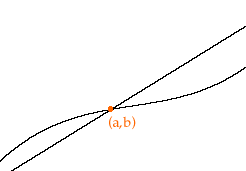Quandaries and Queries Name: senthil Question: sir, between line and curve how can i find intersection point? could you write me the formula and explanation also sir. thanking you Hi Senthil, Lets do it with an example. Suppose that you have the line y = 2x + 3 and the curve y = x2 + 3x + 1 and you want to find any intersection points. What I see in my mind is a curve and a line that intersect, as in the diagram below, where (a,b) is the point of intersection.Since (a,b) is on both graphs, the line and the curve, is satisfies both equations. That is b = 2a + 3 and b = a2 + 3a + 1 Thus a2 + 3a + 1 = b = 2a + 3 Thus if you solve a2 + 3a + 1 = 2a + 3 for a you will find the x-coordinates of any points of intersection. Simplifying this equation I get a2 + a - 2 = 0 which I can factor as (a - 1)(a + 2) = 0 and thus a = 1 or a = -2. If you now substitute these values for x into either either equation you will find the y-coordinates of the two points of intersection. I hope this helps, Penny Go to Math Central